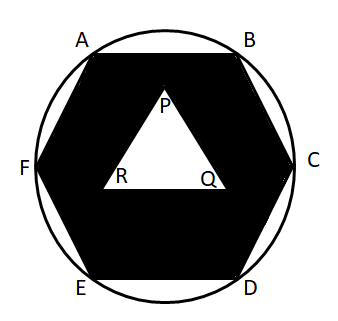# IIFT 2014 Question Paper

Instructions

For the following questions answer them individually

Question 101

# A Boat is being rowed away in still water, from a 210 metres high cliff at the speed of 3 km/hr. What is the approximate time taken for the angle of depression of the cliff at the boat to change from 60 deg. To 45 deg.?Question 102

# X and Y are the two alloys which were made by mixing Zinc and Copper in the ratio 6 : 9 and 7 : 11 respectively. If 40 grams of alloy X and 60 grams of alloy Y are melted and mixed to form another alloy Z, what is the ratio of Zinc and Copper in the new alloy Z?Question 103

# ABCDEF is a regular hexagon of side a and PQR is an equilateral triangle. The area of the shaded portion is X and CD : PQ : : 2 : 1. Find the area of the circle circumscribing the hexagon in terms of X.Question 104

# Ravindra and Rekha got married 10 years ago, their ages were in the ratio of 5 : 4. Today Ravindra’s age is one sixth more than Rekha’s age. After marriage, they had 6 children including a triplet and twins. The age of the triplets, twins and the sixth child is in the ratio of 3 : 2 : 1. What is the largest possible value of the present total age of the family?Question 105

# In the MBA Programme of a B - School, there are two sections A and B. 1/4th of the students in Section A and 4/9th of the students in section B are girls. If two students are chosen at random, one each from section A and Section B as class representative, the probability that exactly one of the students chosen is a girl, is :Question 106

# A milk vendor sells 10 litres of milk from a can containing 40 litres of pure milk to the 1st customer. He then adds 10 litres of water to the milk can. He again sells 10 litres of mixture to the 2nd customer and then adds 10 litres of water to the can. Again he sells 10 litres of mixture to the 3rd customer and then adds 10 litres of water to the can and so on. What amount of pure milk will the 5th customer receive?Question 107

# A ferry carries passengers to Rock of Vivekananda and back from Kanyakumari. The distance of Rock of Vivekananda from Kanyakumari is 100km. One day, the ferry started for Rock of Vivekananda with passengers on board, at a speed of 20 km per hour. After 90 minutes, the crew realized that there is a hole in the ferry and 15 gallons of sea water had already entered the ferry. Sea water is entering the ferry at the rate of 10 gallons per hour. It requires 60 gallons of water to sink the ferry. At what speed should the driver now drive the ferry so that it can reach the Rock of Vivekananda and return back to Kanyakumari just in time before the ferry sinks? (Current of the sea water from Rock of Vivekananda to Kanyakumari is 2km per hour.)Question 108

# The sum of $$1 - \frac{1}{6} + (\frac{1}{6} \times \frac{1}{4})-(\frac{1}{6} \times \frac{1}{4} \times \frac{5}{18})+$$ .... isQuestion 109

# The value of $$\text{log}_{7} \text{log}_{7} \sqrt{7(\sqrt{7\sqrt{7}})}$$Question 110

# A ladder just reaches a window that is 8 metres high above the ground on one side of the street. Keeping one end of the ladder at the same place, the ladder is moved to the other side of the street so as to reach a 12 metre high window. If the ladder is 13 metres long, what is the width of the street?OR